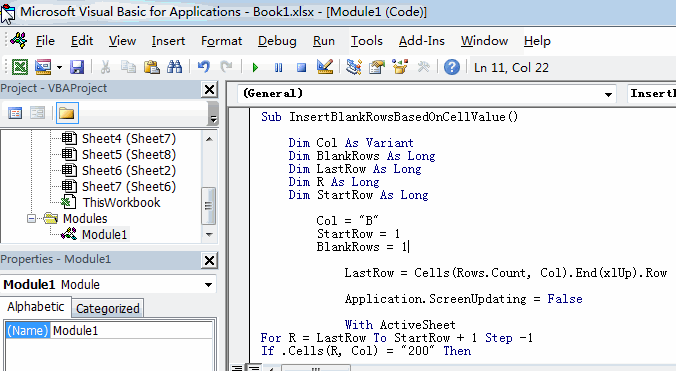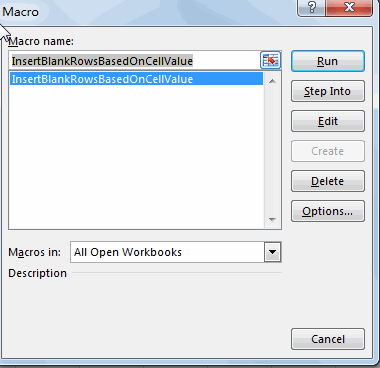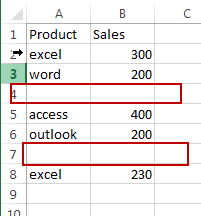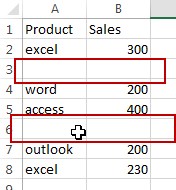# How to Insert Blank Row based on Cell Value in Excel

This post will guide you how to insert a blank row below based on cell value in Excel. How do I auto insert row based on cell value with a VBA Macro in Excel.

## Insert Blank Row Below based on Cell Value

Assuming that you have a list of data in range A1:B6, in which contain sales data. And you want to insert a blank row below based on cell value in Sales column, and if sales value is equal to the certain value, such as: 200, then insert blank row below the certain cell value. How to do it. You can try to use an Excel VBA macro to achieve the result. Here are the steps:

#1 open your excel workbook and then click on “Visual Basic” command under DEVELOPER Tab, or just press “ALT+F11” shortcut.#2 then the “Visual Basic Editor” window will appear.

#3 click “Insert” ->”Module” to create a new module.#4 paste the below VBA code  into the code window. Then clicking “Save” button.```Sub InsertBlankRowsBasedOnCellValue()

Dim Col As Variant
Dim BlankRows As Long
Dim LastRow As Long
Dim R As Long
Dim StartRow As Long

Col = "B"
StartRow = 1
BlankRows = 1

LastRow = Cells(Rows.Count, Col).End(xlUp).Row

Application.ScreenUpdating = False

With ActiveSheet
For R = LastRow To StartRow + 1 Step -1
If .Cells(R, Col) = "200" Then
.Cells(R + 1, Col).EntireRow.Insert Shift:=xlDown
End If
Next R
End With
Application.ScreenUpdating = True

End Sub```

Note: you need to change the value of variable Col as you need, this column contain cell value that you need to base on. and you also need to change the certain cell value 200 as you need .

#5 back to the current worksheet, then run the above excel macro. Click Run button.#6 Let’s see the last result.If you want to insert a blank cell above based on a certain value in another column, you can use the below VBA Macro to achieve the result:

```Sub InsertBlankRowsBasedOnCellValue()

Dim Col As Variant
Dim BlankRows As Long
Dim LastRow As Long
Dim R As Long
Dim StartRow As Long

Col = "B"
StartRow = 1
BlankRows = 1

LastRow = Cells(Rows.Count, Col).End(xlUp).Row

Application.ScreenUpdating = False

With ActiveSheet
For R = LastRow To StartRow + 1 Step -1
If .Cells(R, Col) = "200" Then
.Cells(R, Col).EntireRow.Insert Shift:=xlDown
End If
Next R
End With
Application.ScreenUpdating = True

End Sub```### You might also like:

Sidebar